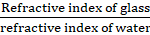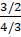### Solve the following examples. If the absolute refractive indices of glass and water are 3/2 and 4/3 respectively, what is the refractive index of glass with respect to water?

Question 8.

Solve the following examples.

If the absolute refractive indices of glass and water are 3/2 and 4/3 respectively, what is the refractive index of glass with respect to water?

Answer:

Refractive Index of glass = 3/2

Refractive index of water = 4/3

So refractive index of glass with respect to water == 9/8

So refractive index of glass with respect to water = 9/8